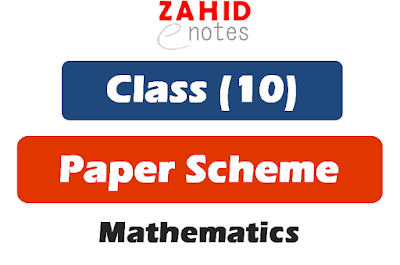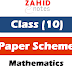This is the paper scheme of mathematics for science group class 10th 2022. The pairing scheme is for the year 2022 and you can download the picture and the image of the scheme. For the 1st time, I have uploaded all the pairing schemes of matric both 9th and 10th in pdf.

# 10th class math pairing scheme 2022

Here is the pairing scheme of maths for 10th class. You can see the following related material for your exams preparation:

## Paper scheme of maths class 10 2022Here is the pairing scheme of 10th class maths in texts form:

MCQs = 15 (1 from chapter 1, 4, 7, 9, 10, 11, 12, each and 2 from chapter 2, 3, 5, 6 each)

### Short Questions

Question No. 2 (6/9)
Chapter 1 = 3
Chapter 2 = 3
Chapter 3 = 3

Question No. 3 (6/9)
Chapter 4 = 2
Chapter 5 = 4
Chapter 6 = 3

Question No. 4 (6/9)
Chapter 7 = 2
Chapter 8 = 1
Chapter 9 = 1
Chapter 10 = 1
Chapter 11 = 2
Chapter 13 = 2

### Long Questions (attempt ANY THREE from FIVE)

Question No. 5
Chapter 1 = one part
Chapter 2 = one part

Question No. 6
Chapter 3 = one part
Chapter 4 = one part

Question No. 7
Chapter 5 = one part
Chapter 6 = one part

Questions No.8
Chapter 7 = one part
Chapter 13 = one part

Questions No.9 (compulsory question)
Chapter 9 OR chapter 12= 1 question

#### You may like to see:

1. 10th class all schemes 2022 pdf
10th class maths pairing scheme 2022Reviewed by Saif Ullah ZahidonMarch 07, 2022Rating: 5

1.ya 2021 ki ha

2.Please share the 2021 ki bi snd kr dyia.

3.Yeah theek hia kiya